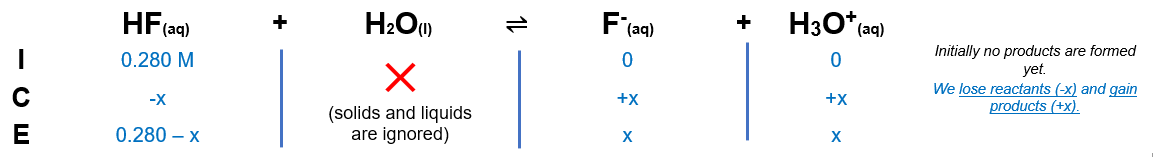# Problem: Determine the pH of an HF solution of each of the following concentrations. In which cases can you not make the simplifying assumption that x is small? (Ka for HF is 6.8 x 10-4.)0.280 M

###### FREE Expert Solution

We are asked to determine the pH of an HF solution.

Step 1. ICE chartStep 2. Calculate x

95% (31 ratings)###### Problem Details

Determine the pH of an HF solution of each of the following concentrations. In which cases can you not make the simplifying assumption that x is small? (Ka for HF is 6.8 x 10-4.)

0.280 M

Frequently Asked Questions

What scientific concept do you need to know in order to solve this problem?

Our tutors have indicated that to solve this problem you will need to apply the Weak Acids concept. If you need more Weak Acids practice, you can also practice Weak Acids practice problems.

What professor is this problem relevant for?

Based on our data, we think this problem is relevant for Professor Hoeger's class at UCSD.